# Python Tuple: The Complete Guide

0
204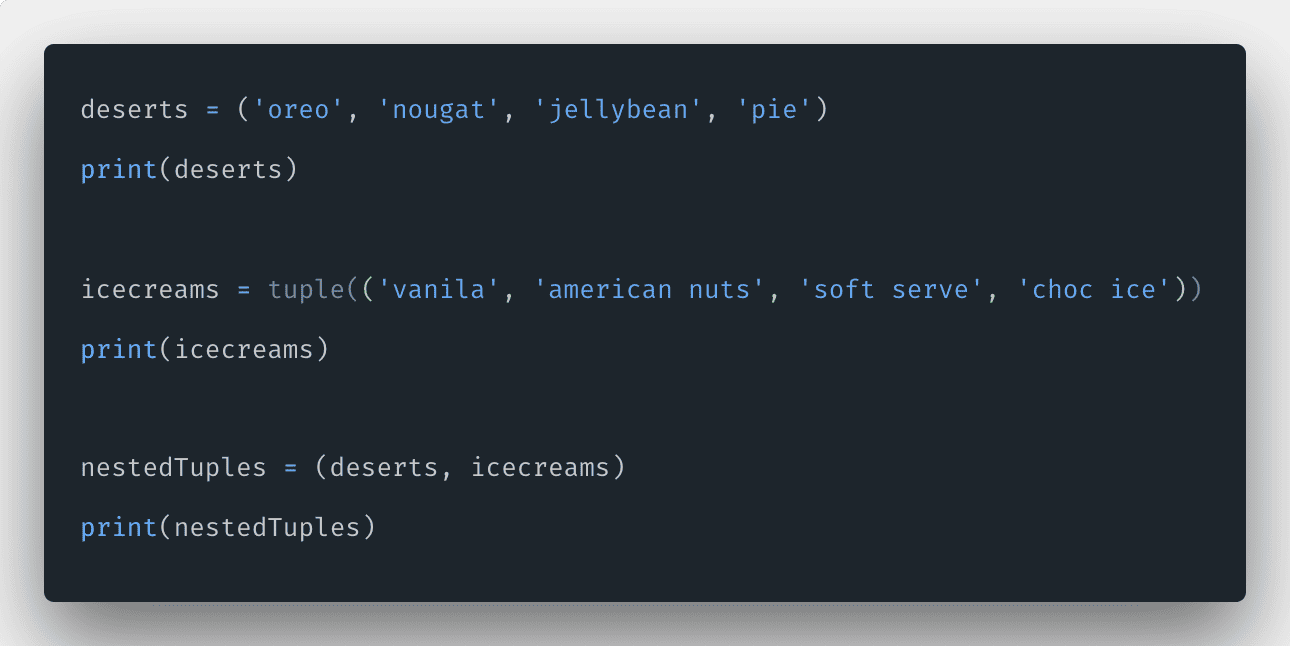In Python programming language, the tuple is similar to a list, with the difference being that we cannot change the items of a tuple once assigned, whereas, in the list, items can be modified. The Tuple is a collection of Python objects separated by commas.

## Python Tuple

A tuple is a built-in Python collection that is ordered and unchangeable. Python tuples are written with round brackets. Since tuples are immutable, iterating through a tuple is faster than a list. So there is a slight performance boost.

If you have data that doesn’t change in time, implementing it as a tuple will guarantee that it remains write-protected.

`deserts = ('oreo', 'cupcake', 'nougat', 'jellybean', 'pie')`

Above is a simple example of Tuple in Python language.

## How to Create a Tuple in Python

To create a tuple in Pythonplace all the elements inside the parentheses (), separated by a comma. The parentheses are optional but are a good practice to write them. A tuple can have any number of items, and they may be of different types.

Let us see the following example of different types of items in one tuple.

```// app.py

deserts = ('oreo', 1, True, ['Android', 'iOS'], 'pie')
print(deserts)```

In the above example, we have taken string, integer, and list datatype to create a tuple, and it is a valid tuple.

Let us write a tuple without parentheses.

```deserts = 'oreo', 1, True, ['Android', 'iOS'], 'pie'
print(deserts)```

The above code is also valid, but it is not the best practice to write. That is why we add the parentheses around the items.

## How to access tuple elements in Python

To access tuple elements in Python, refer to the index number inside square brackets.

Let us see the following example.

```// app.py

deserts = ('oreo', 1, True, ['Android', 'iOS'], 'pie')
print(deserts)```

See the following output.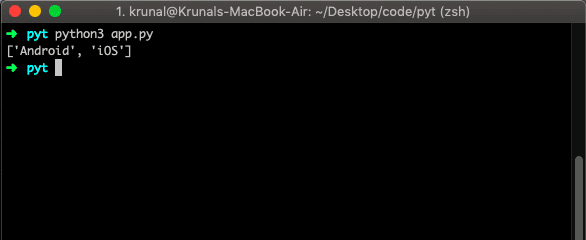## How to loop through a Tuple in Python

To loop through a tuple in Python, use the for loop. A for loop is used for iterating over a sequence. The for loop does not require an indexing variable to set beforehand.

Let us see the below example.

```# app.py

deserts = ('oreo', 'nougat', 'jellybean', 'pie')
for item in deserts:
print(item)```

The output is the following.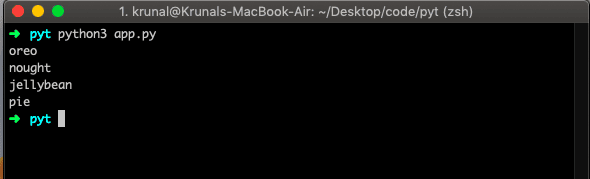## Try To Change Tuple Values

Let us change the values in a tuple and see the result.

```# app.py

deserts = ('oreo', 'nougat', 'jellybean', 'pie')
deserts = 'kitkat'
print(deserts)```

I am using Visual Studio Code and have installed a Python extension called pylint. That is why my editor throws me an error saying that ‘deserts’ does not support item assignment. Unfortunately, that means we can not modify it. But still, let us run the file and see the output in the console.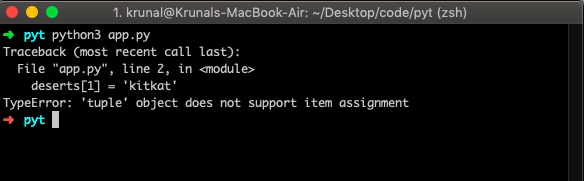## Python tuple length

To find a length of a tuple in Python, use the len() function. The len() method returns how many elements a tuple has.

```# app.py

deserts = ('oreo', 'nougat', 'jellybean', 'pie')
print(len(deserts))```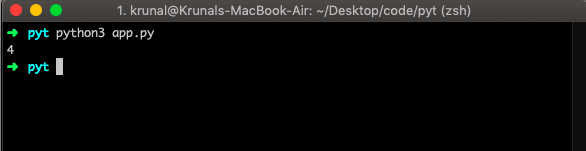## How to remove an element in Tuple

A tuple is unchangeable, so we can’t remove single or multiple elements, but we can delete the entire tuple.

See the below example.

```// app.py

deserts = ('oreo', 'nougat', 'jellybean', 'pie')
del deserts
print(deserts)```

We have used the del keyword to delete the entire tuple. So the above code’s output will get an error because the tuple is already removed. So there is nothing to print because the compiler does not find any deserts tuple. So it throws an error.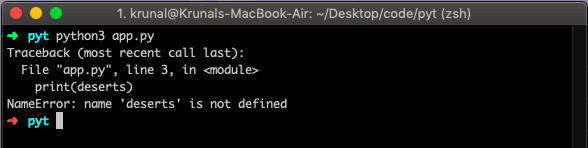## The tuple() Constructor

We can also use the tuple() constructor to make a tuple.

```# app.py

icecreams = tuple(('vanila', 'american nuts', 'soft serve', 'choc ice'))
print(icecreams)```

The output will be the same.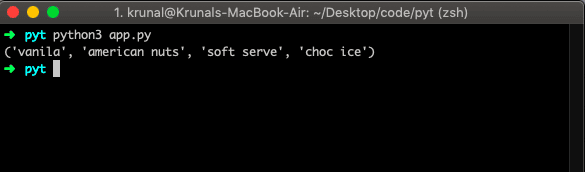## Basic Tuples Operations

Tuples respond to the + and * operators much like strings in Python; they mean concatenation and repetition here, except that a result is a new tuple, not a string.

Python Expression Results Description
len((1, 2, 3, 4, 5, 6)) 6 Length
(11, 21, 31) + (41, 51, 61) (11, 21, 31, 41, 51, 61) Concatenation
(‘App’,) * 4 (‘App’, ‘App’, ‘App’, ‘App’) Repetition
21 in (11, 21, 31) True Membership
for z in (11, 21, 31): print(z), 11 21 31 Iteration

## Nesting of Tuples

Let us see the following example of nested tuples.

```# app.py

deserts = ('oreo', 'nougat', 'jellybean', 'pie')

icecreams = tuple(('vanila', 'american nuts', 'soft serve', 'choc ice'))

nestedTuples = (deserts, icecreams)
print(nestedTuples)```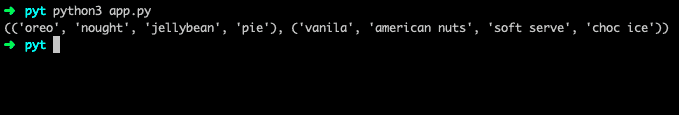That’s it for this tutorial.

This site uses Akismet to reduce spam. Learn how your comment data is processed.